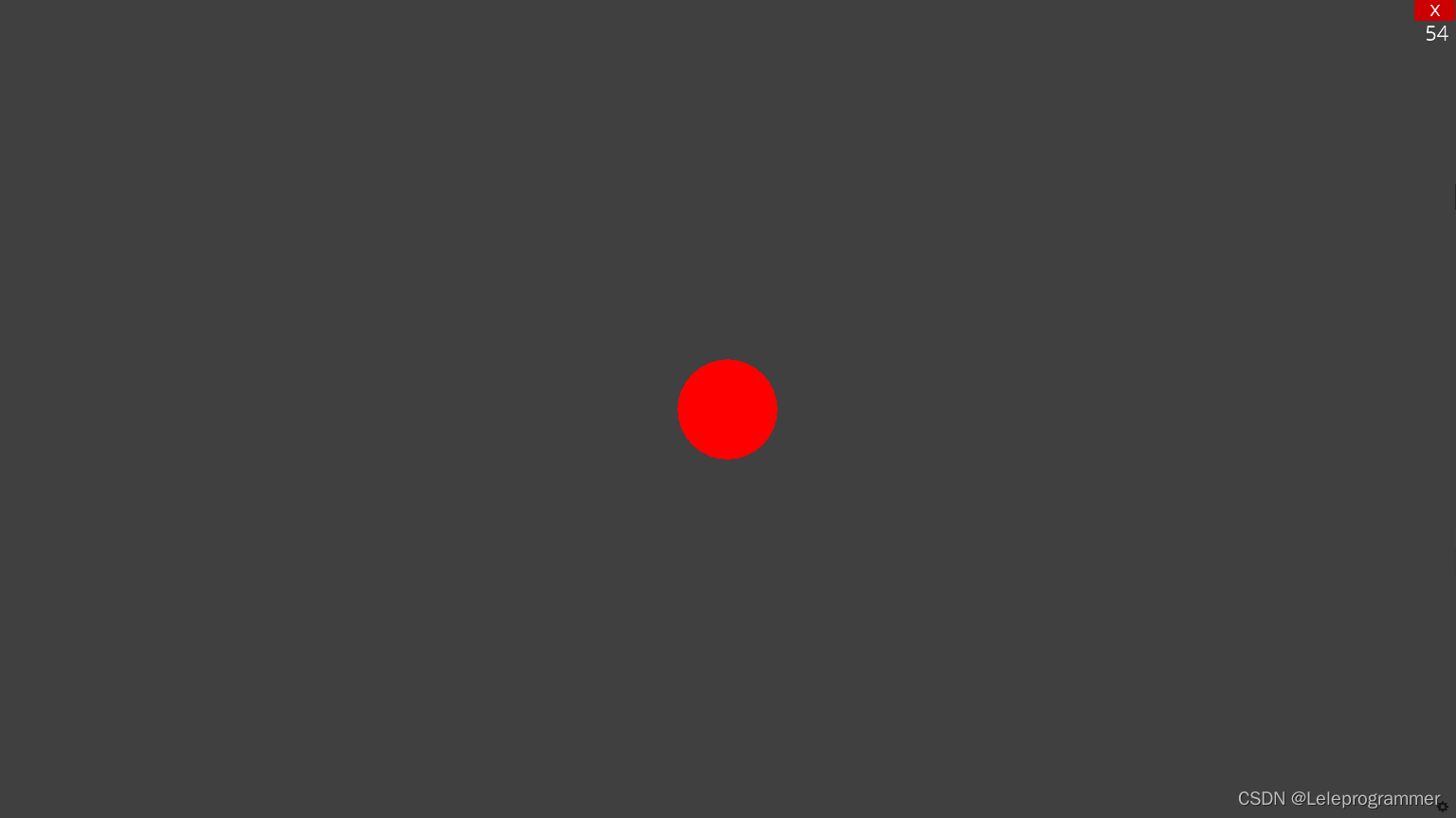# 手把手教你用Python编一个《我的世界》 1. 认识Ursina并学会绘制立体图形

Python有一个不错的3D引擎——Ursina

Ursina官网：www.ursinaengine.org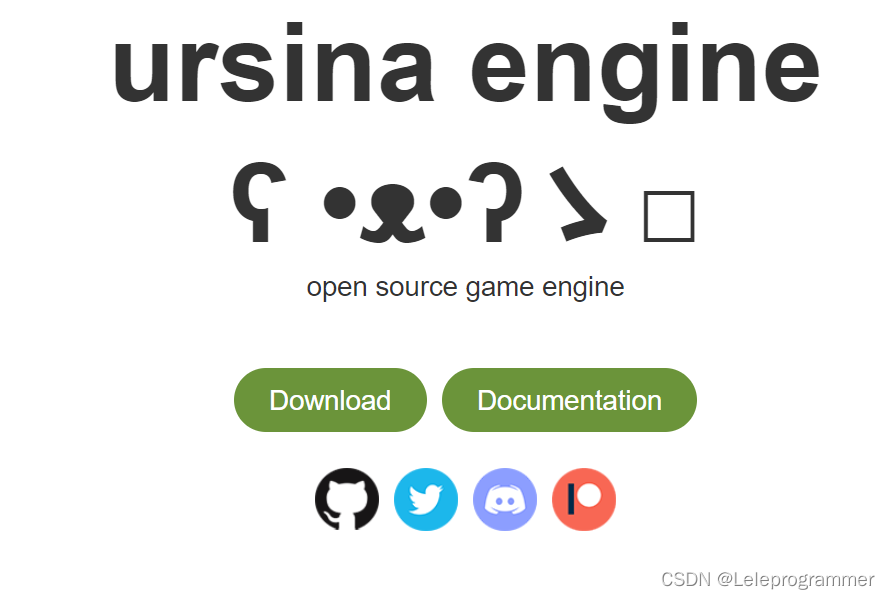# 编写第一个程序

``from ursina import *``

``app=Ursina()``

``app.run()``

``````from ursina import *

app=Ursina()

app.run()``````

# 绘制实体长方体

Entity()

``````from ursina import *

app=Ursina()

cube=Entity(model="cube")

app.run()``````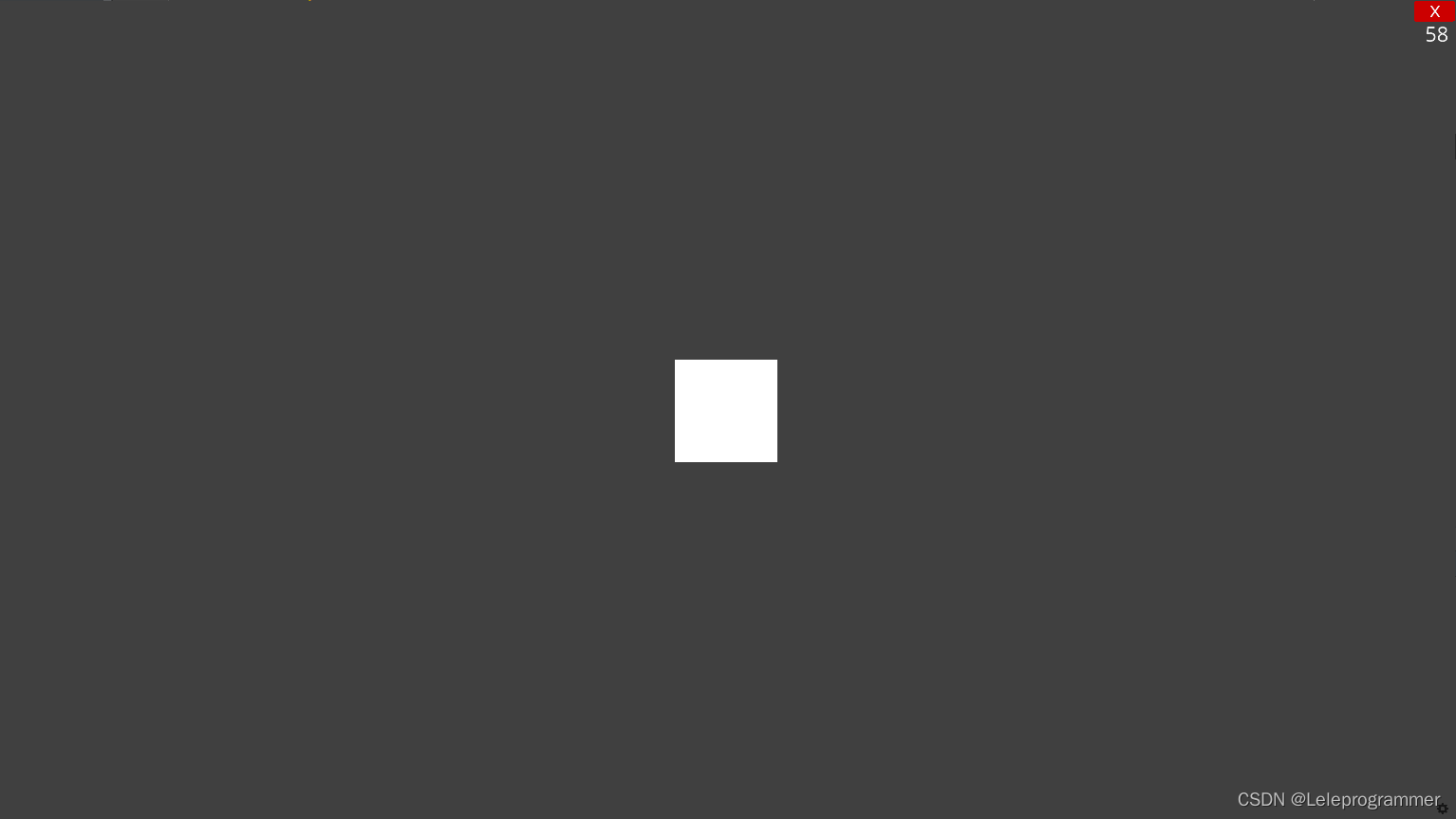# 对实体进行同样比例的放大和缩小

``````from ursina import *

app=Ursina()

cube=Entity(model="cube",scale=2)

app.run()``````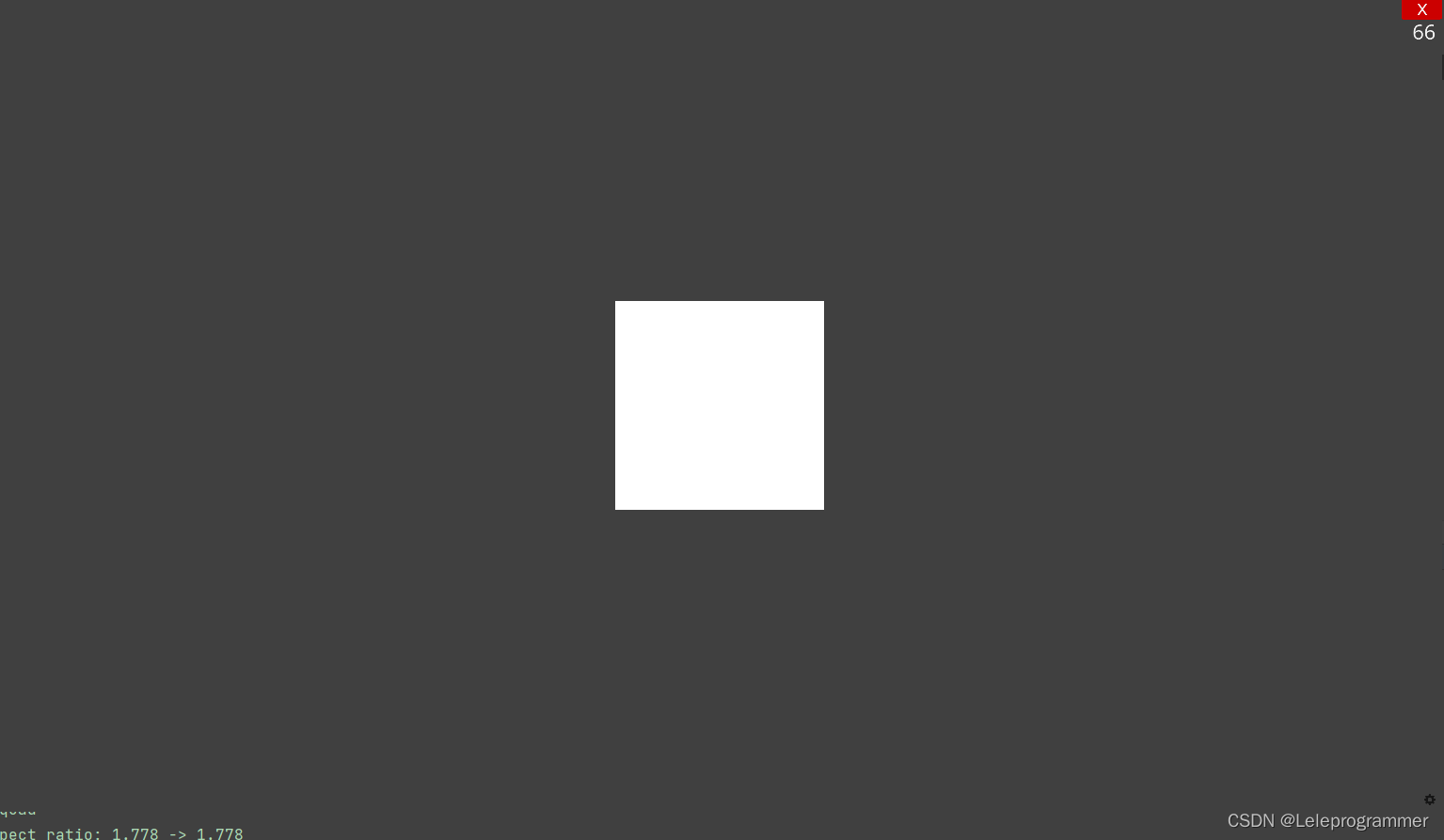# 对实体进行任意放大

``````from ursina import *

app=Ursina()

cube=Entity(model="cube",scale_x=2)

app.run()``````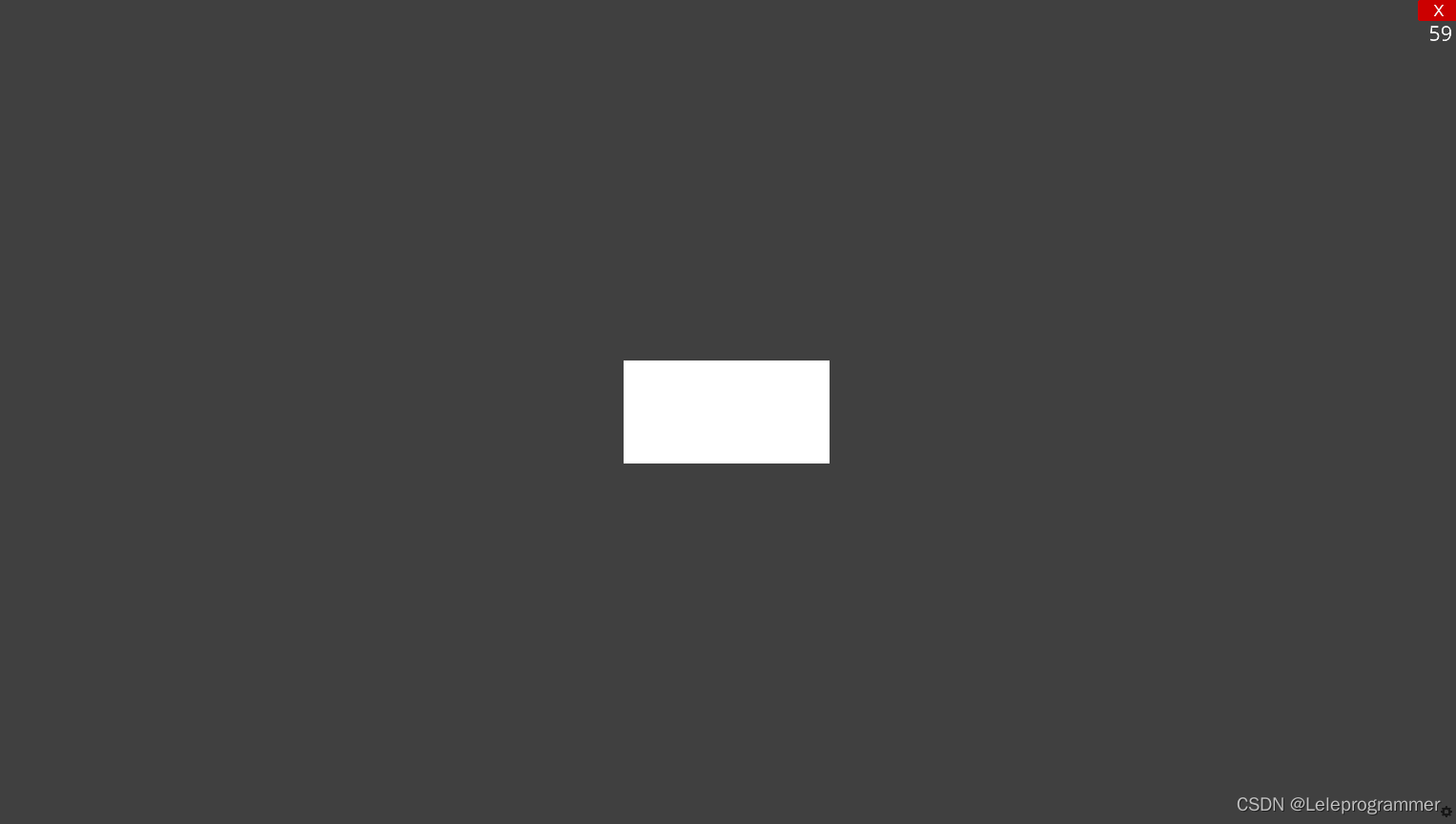``````from ursina import *

app=Ursina()

cube=Entity(model="cube",scale_y=2)

app.run()``````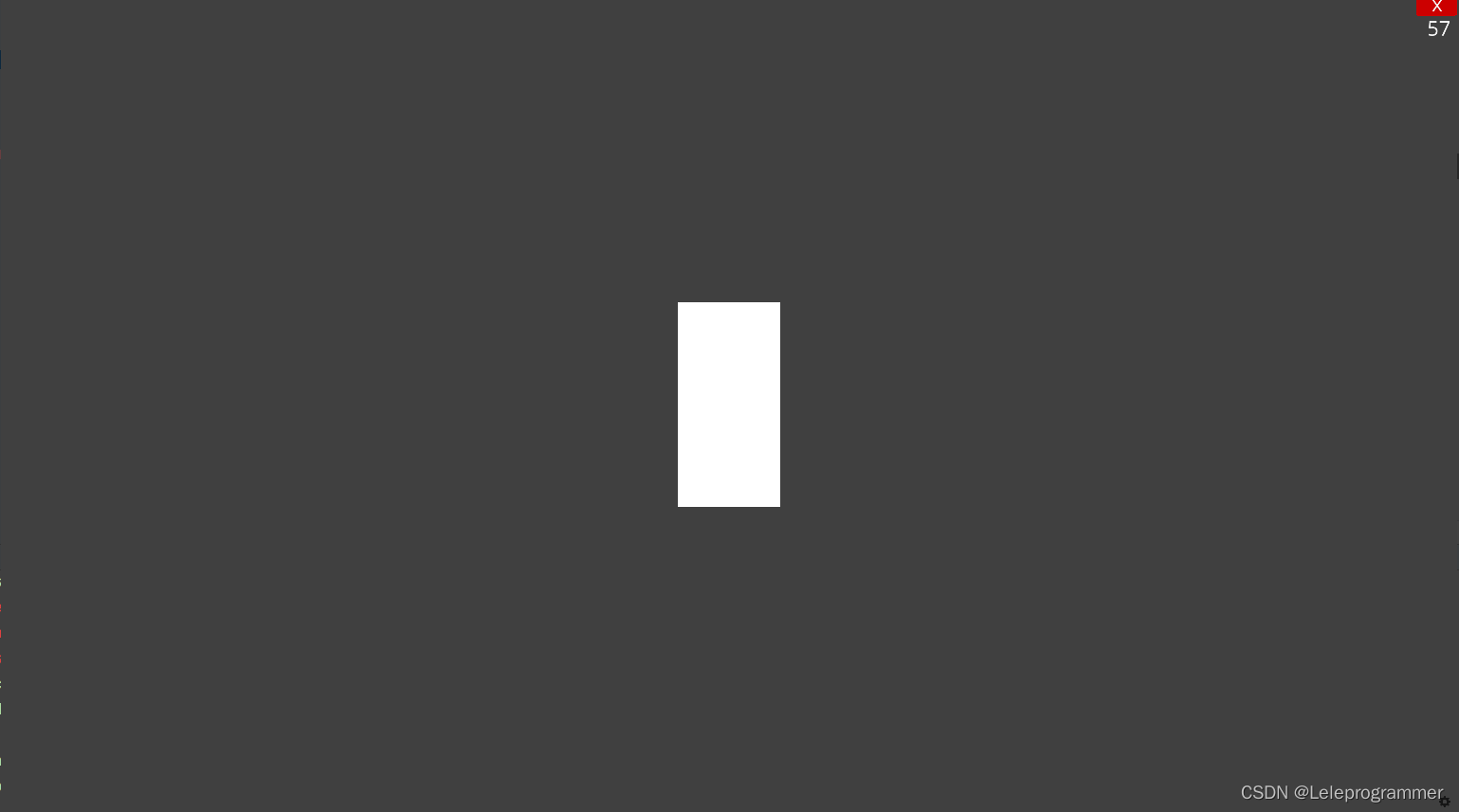scale参数还可以传入一个元组，格式为(float,float,float)，表示xyz边各放大不同的倍数，代码示例如下：

``````from ursina import *

app=Ursina()

cube=Entity(model="cube",scale=(2,3,4))

app.run()``````

# 绘制球体

``````from ursina import *

app=Ursina()

sphere=Entity(model="sphere")

app.run()``````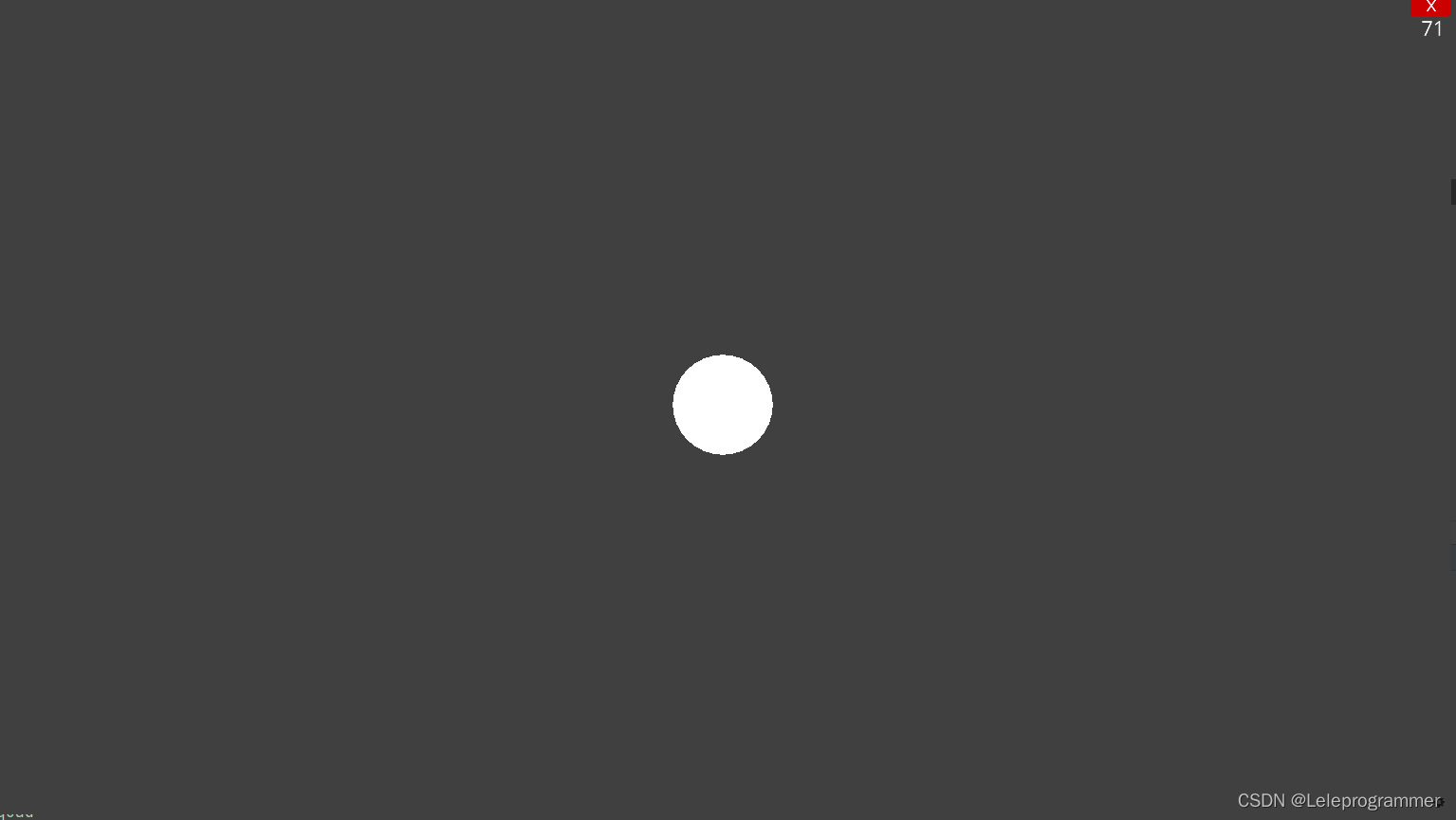# 为你的实体上色

Ursina自带color模块，一些常用的颜色可以通过 color.颜色名 进行使用，也可以用color.rgb()函数传入rgb或rgba的数值，示例：

``````from ursina import *

app=Ursina()

sphere=Entity(model="sphere",color=color.red)

app.run()``````
``````from ursina import *

app=Ursina()

sphere=Entity(model="sphere",color=color.rgb(255,0,0))

app.run()``````